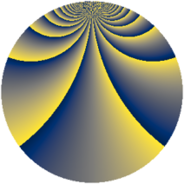# Properties

 Label 2366.2.bjLevel $2366$ Weight $2$ Character orbit 2366.bj Rep. character $\chi_{2366}(29,\cdot)$ Character field $\Q(\zeta_{39})$ Dimension $2160$ Sturm bound $728$

# Related objects

## Defining parameters

 Level: $$N$$ $$=$$ $$2366 = 2 \cdot 7 \cdot 13^{2}$$ Weight: $$k$$ $$=$$ $$2$$ Character orbit: $$[\chi]$$ $$=$$ 2366.bj (of order $$39$$ and degree $$24$$) Character conductor: $$\operatorname{cond}(\chi)$$ $$=$$ $$169$$ Character field: $$\Q(\zeta_{39})$$ Sturm bound: $$728$$

## Dimensions

The following table gives the dimensions of various subspaces of $$M_{2}(2366, [\chi])$$.

Total New Old
Modular forms 8832 2160 6672
Cusp forms 8640 2160 6480
Eisenstein series 192 0 192

## Trace form

 $$2160q - 2q^{2} + 90q^{4} - 4q^{5} + 4q^{8} + 90q^{9} + O(q^{10})$$ $$2160q - 2q^{2} + 90q^{4} - 4q^{5} + 4q^{8} + 90q^{9} + 6q^{10} - 4q^{11} + 6q^{13} + 8q^{14} + 56q^{15} + 90q^{16} + 6q^{17} + 12q^{18} - 16q^{19} + 2q^{20} - 8q^{21} + 48q^{22} + 12q^{23} - 200q^{25} - 10q^{26} - 24q^{27} + 10q^{29} - 252q^{30} + 96q^{31} - 2q^{32} + 32q^{33} + 12q^{34} - 4q^{35} + 90q^{36} + 6q^{37} - 32q^{38} + 52q^{39} + 66q^{40} + 14q^{41} - 4q^{42} + 8q^{43} + 8q^{44} + 234q^{45} + 12q^{46} - 36q^{47} + 90q^{49} - 4q^{50} + 204q^{51} + 38q^{53} - 12q^{54} + 220q^{55} - 4q^{56} + 180q^{57} + 54q^{58} + 16q^{59} + 96q^{60} + 18q^{61} + 12q^{62} + 4q^{63} - 180q^{64} + 18q^{65} - 16q^{66} + 64q^{67} + 6q^{68} + 12q^{69} - 6q^{72} + 36q^{73} + 64q^{74} + 40q^{75} - 16q^{76} - 12q^{78} - 64q^{79} + 2q^{80} + 70q^{81} + 18q^{82} - 496q^{83} + 4q^{84} + 112q^{85} - 24q^{86} + 92q^{87} - 4q^{88} + 492q^{89} - 84q^{90} - 4q^{91} - 24q^{92} + 300q^{93} + 112q^{94} - 204q^{95} - 12q^{97} - 2q^{98} - 40q^{99} + O(q^{100})$$

## Decomposition of $$S_{2}^{\mathrm{new}}(2366, [\chi])$$ into newform subspaces

The newforms in this space have not yet been added to the LMFDB.

## Decomposition of $$S_{2}^{\mathrm{old}}(2366, [\chi])$$ into lower level spaces

$$S_{2}^{\mathrm{old}}(2366, [\chi]) \cong$$ $$S_{2}^{\mathrm{new}}(169, [\chi])$$$$^{\oplus 4}$$$$\oplus$$$$S_{2}^{\mathrm{new}}(338, [\chi])$$$$^{\oplus 2}$$$$\oplus$$$$S_{2}^{\mathrm{new}}(1183, [\chi])$$$$^{\oplus 2}$$